# 图像处理中小波变换滤波

## 一、图像处理之均值滤波，高斯滤波(高斯模糊)，中值滤波，双边滤波

2018-08-30 10:19:31 csdnforyou 阅读数 22756
• ###### MATLAB图像处理

介绍MATLAB提供的图像处理功能，包括图像基础知识、图像合成、空间变换、邻域和块处理、局部滤波、正交变换、数学形态学、图像分析、图像增强、图像恢复、图像分割、图像配准和图像三维重建等内容。

47课时 342分钟 232人学习 苏金明
免费试看

### 1. 滤波器介绍

1）空间域指图像本身，空域变换直接对图像中的像素进行操作。

2）图像变换是将图像从空间域变换到某变换域（如 傅立叶变换中的频率域）的数学变换，在变换域 中进行处理，然后通过反变换把处理结果返回到空间域。

3）图像在空域上具有很强的相关性，借助于正交变 换可使在空域的复杂计算转换到频域后得到简化

4）借助于频域特性的分析，将更有利于获得图像的 各种特性和进行特殊处理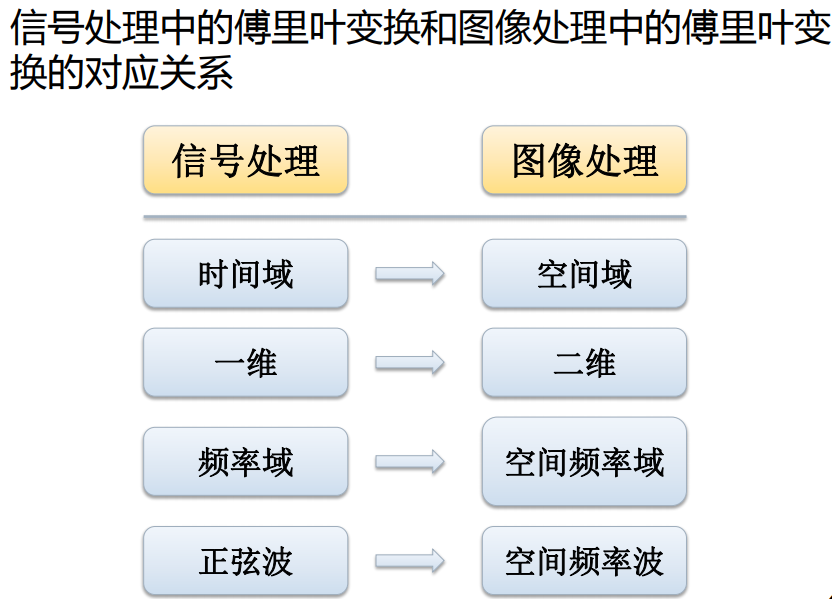## 2、理论知识：

滤波的意思就是对原图像的每个像素周围一定范围内的像素进行运算，运算的范围就称为掩膜。而运算就分两种了，如果运算只是对各像素灰度值进行简单处理（如乘一个权值）最后求和，就称为线性滤波；而如果对像素灰度值的运算比较复杂，而不是最后求和的简单运算，则是非线性滤波；如求一个像素周围3x3范围内最大值、最小值、中值、均值等操作都不是简单的加权，都属于非线性滤波。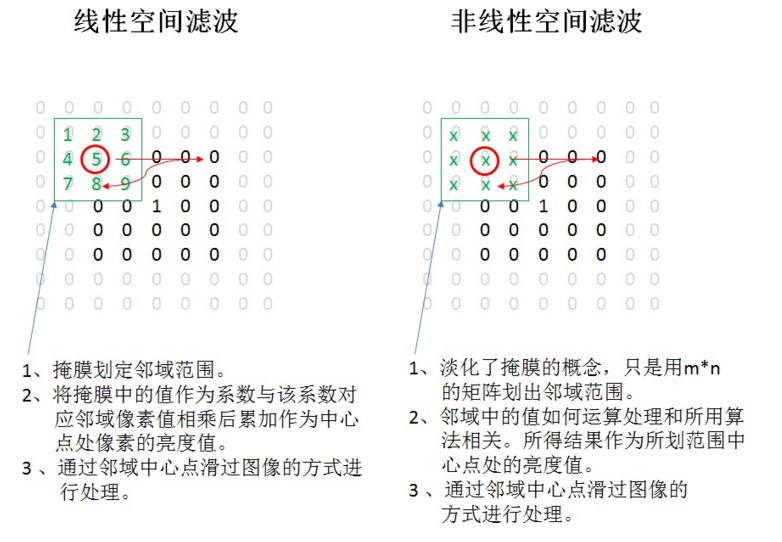1、线性滤波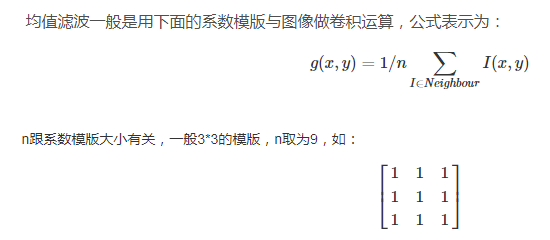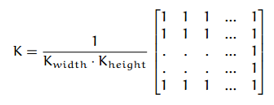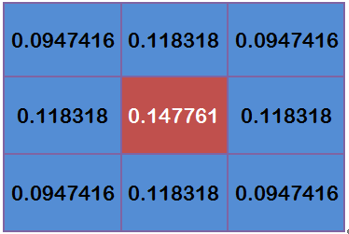# 详细请参考：数字图像处理之椒盐噪声和中值滤波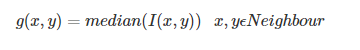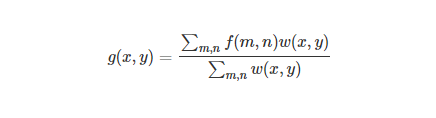w(x,y)为加权系数，取决于定义域核和值域核的乘积。

1）均值模糊无法克服边缘像素信息丢失的缺陷，原因是均值滤波是基于平均权重的。

2）高斯模糊部分克服了该缺陷，但无法完全避免，因为没有考虑像素值的不同。

3）高斯双边模糊-是边缘保留额滤波方法，避免了边缘信息丢失，保留了图像轮廓不变。

### 3. 实验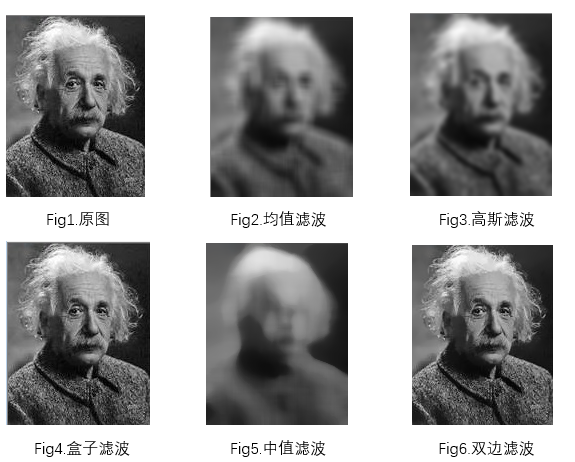### 4. C++实现

4.1均值滤波

static void exchange(int& a, int& b)
{
int t = 0;
t = a;
a = b;
b = t;
}

static void bubble_sort(int* K, int lenth)
{
for (int i = 0; i < lenth; i++)
for (int j = i + 1; j < lenth; j++)
{
if (K[i]>K[j])
exchange(K[i], K[j]);
}
}
///产生二维的高斯内核
static cv::Mat generate_gassian_kernel(double u, double sigma, cv::Size size)
{
int width = size.width;
int height = size.height;
cv::Mat gassian_kernel(cv::Size(width, height), CV_64FC1);
double sum = 0;
double sum_sum = 0;
for (int i = 0; i < width; i++)
for (int j = 0; j < height; j++)
{
sum = 1.0 / 2.0 / CV_PI / sigma / sigma * exp(-1.0 * ((i - width / 2)*(i - width / 2) + (j - width / 2)*(j - width / 2)) / 2.0 / sigma / sigma);
sum_sum += sum;
gassian_kernel.ptr<double>(i)[j] = sum;
}
for (int i = 0; i < width; i++)
for (int j = 0; j < height; j++)
{
gassian_kernel.ptr<double>(i)[j] /= sum_sum;
}
return gassian_kernel;
}
///均值滤波
void lmt_main_blur(cv::Mat& img_in, cv::Mat& img_out, int kernel_size)
{
img_out = img_in.clone();
cv::Mat mat1;
cv::copyMakeBorder(img_in, mat1, kernel_size, kernel_size, kernel_size, kernel_size, cv::BORDER_REPLICATE);

int cols = mat1.cols;
int rows = mat1.rows;
int channels = img_out.channels();
const uchar* const pt = mat1.ptr<uchar>(0);
uchar* pt_out = img_out.ptr<uchar>(0);

for (int i = kernel_size; i < rows - kernel_size; i++)
{
for (int j = kernel_size; j < cols - kernel_size; j++)
{
if (channels == 1)
{
long long int sum_pixel = 0;
for (int m = -1 * kernel_size; m < kernel_size; m++)
for (int n = -1 * kernel_size; n < kernel_size; n++)
{
sum_pixel += pt[(i + m)*cols + (j + n)];
}
img_out.ptr<uchar>(i - kernel_size)[j - kernel_size] = (double)sum_pixel / (kernel_size*kernel_size * 4);
}
else if (channels == 3)
{
long long int sum_pixel = 0;
long long int sum_pixel1 = 0;
long long int sum_pixel2 = 0;
for (int m = -1 * kernel_size; m < kernel_size; m++)
for (int n = -1 * kernel_size; n < kernel_size; n++)
{
sum_pixel += pt[((i + m)*cols + (j + n))*channels + 0];
sum_pixel1 += pt[((i + m)*cols + (j + n))*channels + 1];
sum_pixel2 += pt[((i + m)*cols + (j + n))*channels + 2];
}
img_out.ptr<uchar>(i - kernel_size)[(j - kernel_size)*channels + 0] = (double)sum_pixel / (double)(kernel_size*kernel_size * 4);
img_out.ptr<uchar>(i - kernel_size)[(j - kernel_size)*channels + 1] = (double)sum_pixel1 / (double)(kernel_size*kernel_size * 4);
img_out.ptr<uchar>(i - kernel_size)[(j - kernel_size)*channels + 2] = (double)sum_pixel2 / (double)(kernel_size*kernel_size * 4);
}
}
}

}
///中值滤波
void lmt_median_blur(cv::Mat& img_in, cv::Mat& img_out, int kernel_size)
{
img_out = img_in.clone();
cv::Mat mat1;
cv::copyMakeBorder(img_in, mat1, kernel_size, kernel_size, kernel_size, kernel_size, cv::BORDER_REPLICATE);

int cols = mat1.cols;
int rows = mat1.rows;
int channels = img_out.channels();

cv::Mat mat;
cv::Mat mat_out;
cv::split(mat1, mat);
cv::split(img_out, mat_out);
for (int k = 0; k < 3; k++)
{
const uchar* const pt = mat[k].ptr<uchar>(0);
uchar* pt_out = mat_out[k].ptr<uchar>(0);
for (int i = kernel_size; i < rows - kernel_size; i++)
{
for (int j = kernel_size; j < cols - kernel_size; j++)
{
long long int sum_pixel = 0;
int* K = new int[kernel_size*kernel_size * 4];
int ker_num = 0;
for (int m = -1 * kernel_size; m < kernel_size; m++)
for (int n = -1 * kernel_size; n < kernel_size; n++)
{
K[ker_num] = pt[(i + m)*cols + (j + n)];
ker_num++;
}
bubble_sort(K, ker_num);
mat_out[k].ptr<uchar>(i - kernel_size)[j - kernel_size] = K[ker_num / 2];
}
}
}
cv::merge(mat_out, 3, img_out);
}
///高斯滤波
void lmt_gaussian_blur(cv::Mat& img_src, cv::Mat& img_dst, cv::Size kernel_size)
{
img_dst = cv::Mat(cv::Size(img_src.cols, img_src.rows), img_src.type());
int cols = img_src.cols;
int rows = img_src.rows;
int channels = img_src.channels();
cv::Mat gassian_kernel = generate_gassian_kernel(0, 1, kernel_size);
int width = kernel_size.width / 2;
int height = kernel_size.height / 2;
for (int i = height; i < rows - height; i++)
{
for (int j = width; j < cols - width; j++)
{
for (int k = 0; k < channels; k++)
{
double sum = 0.0;
for (int m = -height; m <= height; m++)
{
for (int n = -width; n <= width; n++)
{
sum += (double)(img_src.ptr<uchar>(i + m)[(j + n)*channels + k]) * gassian_kernel.ptr<double>(height + m)[width + n];
}
}
if (sum > 255.0)
sum = 255;
if (sum < 0.0)
sum = 0;
img_dst.ptr<uchar>(i)[j*channels + k] = (uchar)sum;
}
}
}

}
///双边滤波
void lmt_bilateral_filter(cv::Mat& img_in, cv::Mat& img_out, const int r, double sigma_d, double sigma_r)
{
int i, j, m, n, k;
int nx = img_in.cols, ny = img_in.rows, m_nChannels = img_in.channels();
const int w_filter = 2 * r + 1; // 滤波器边长

double gaussian_d_coeff = -0.5 / (sigma_d * sigma_d);
double gaussian_r_coeff = -0.5 / (sigma_r * sigma_r);
double  **d_metrix = new double *[w_filter];
for (int i = 0; i < w_filter; ++i)
d_metrix[i] = new double[w_filter];

double r_metrix;  // similarity weight
img_out = cv::Mat(img_in.size(),img_in.type());
uchar* m_imgData = img_in.ptr<uchar>(0);
uchar* m_img_outData = img_out.ptr<uchar>(0);
// copy the original image
double* img_tmp = new double[m_nChannels * nx * ny];
for (i = 0; i < ny; i++)
for (j = 0; j < nx; j++)
for (k = 0; k < m_nChannels; k++)
{
img_tmp[i * m_nChannels * nx + m_nChannels * j + k] = m_imgData[i * m_nChannels * nx + m_nChannels * j + k];
}

// compute spatial weight
for (i = -r; i <= r; i++)
for (j = -r; j <= r; j++)
{
int x = j + r;
int y = i + r;

d_metrix[y][x] = exp((i * i + j * j) * gaussian_d_coeff);
}

// compute similarity weight
for (i = 0; i < 256; i++)
{
r_metrix[i] = exp(i * i * gaussian_r_coeff);
}

// bilateral filter
for (i = 0; i < ny; i++)
for (j = 0; j < nx; j++)
{
for (k = 0; k < m_nChannels; k++)
{
double weight_sum, pixcel_sum;
weight_sum = pixcel_sum = 0.0;

for (m = -r; m <= r; m++)
for (n = -r; n <= r; n++)
{
if (m*m + n*n > r*r) continue;

int x_tmp = j + n;
int y_tmp = i + m;

x_tmp = x_tmp < 0 ? 0 : x_tmp;
x_tmp = x_tmp > nx - 1 ? nx - 1 : x_tmp;   // 边界处理，replicate
y_tmp = y_tmp < 0 ? 0 : y_tmp;
y_tmp = y_tmp > ny - 1 ? ny - 1 : y_tmp;

int pixcel_dif = (int)abs(img_tmp[y_tmp * m_nChannels * nx + m_nChannels * x_tmp + k] - img_tmp[i * m_nChannels * nx + m_nChannels * j + k]);
double weight_tmp = d_metrix[m + r][n + r] * r_metrix[pixcel_dif];  // 复合权重

pixcel_sum += img_tmp[y_tmp * m_nChannels * nx + m_nChannels * x_tmp + k] * weight_tmp;
weight_sum += weight_tmp;
}

pixcel_sum = pixcel_sum / weight_sum;
m_img_outData[i * m_nChannels * nx + m_nChannels * j + k] = (uchar)pixcel_sum;

} // 一个通道

} // END ALL LOOP
for (i = 0; i < w_filter; i++)
delete[] d_metrix[i];
delete[] d_metrix;
}

### 5. Opencv API实现

opencv相关函数简介：

src待滤波图像

dst滤波后图像

d滤波器半径

sigmaColor滤波器值域的sigma

sigmaSpace滤波器空间域的sigma

borderType边缘填充方式 BORDER_REPLICATE BORDER_REFLECT BORDER_DEFAULT BORDER_REFLECT_101BORDER_TRANSPARENT BORDER_ISOLATED

src待滤波图像

dst滤波后图像

ksize 均值滤波器的大小

Piont（-1，-1）指中心

anchor均值滤波器的锚点也就是模板移动点

borderType边缘填充方式 BORDER_REPLICATE BORDER_REFLECT BORDER_DEFAULT BORDER_REFLECT_101BORDER_TRANSPARENT BORDER_ISOLATED

src待滤波图像

dst滤波后图像

ksize 高斯滤波器的大小Size（x,y）,x和y必须是整数且是奇数。

sigmaX 高斯滤波器的x方向的滤波器高斯sigma

sigmaY 高斯滤波器的y方向的滤波器高斯sigma

borderType边缘填充方式 BORDER_REPLICATE BORDER_REFLECT BORDER_DEFAULT BORDER_REFLECT_101BORDER_TRANSPARENT BORDER_ISOLATED

src待滤波图像

dst滤波后图像

ksize 中值滤波器的大小

void bilateral_filter_show(void)
{
if (mat1.empty())
return;
cv::imshow("原图像", mat1);
cv::imshow("原始彩色图像", src);
std::cout << "channel = " << mat1.channels() << std::endl;

cv::Mat mat3;
cv::bilateralFilter(src, mat3, 5, 50, 50,cv::BORDER_DEFAULT);
cv::imshow("opencv给出的双边滤波器", mat3);
cv::Mat mat4;
cv::blur(src, mat4, cv::Size(3, 3));
cv::imshow("均值滤波", mat4);
cv::Mat mat5;
cv::GaussianBlur(src, mat5, cv::Size(5, 5), 1,1);
cv::imshow("高斯滤波器", mat5);
cv::Mat mat6;
cv::medianBlur(src, mat6, 3);
cv::imshow("中值滤波", mat6);
cv::Mat mat7;
lmt_gaussian_blur(src, mat7, cv::Size(5, 5));
cv::imshow("my gaussian image",mat7);

cv::waitKey(0);
}

# 高斯、中值、均值、双边滤波的效果

#include "cv.h"
#include "highgui.h"
#include <iostream>

using namespace std;
using namespace cv;

int main(int argc, char* argv[])
{
Mat dst;

//参数是按顺序写的

//高斯滤波
//src:输入图像
//dst:输出图像
//Size(5,5)模板大小，为奇数
//x方向方差
//Y方向方差
GaussianBlur(src,dst,Size(5,5),0,0);
imwrite("gauss.jpg",dst);

//中值滤波
//src:输入图像
//dst::输出图像
//模板宽度，为奇数
medianBlur(src,dst,3);
imwrite("med.jpg",dst);

//均值滤波
//src:输入图像
//dst:输出图像
//模板大小
//Point(-1,-1):被平滑点位置，为负值取核中心
blur(src,dst,Size(3,3),Point(-1,-1));
imwrite("mean.jpg",dst);

//双边滤波
//src:输入图像
//dst:输入图像
//滤波模板半径
//颜色空间标准差
//坐标空间标准差
bilateralFilter(src,dst,5,10.0,2.0);//这里滤波没什么效果，不明白
imwrite("bil.jpg",dst);

waitKey();

return 0;
}

## 图像处理的几种滤波

2018-06-19 01:57:50 hellocsz 阅读数 14833
• ###### MATLAB图像处理

介绍MATLAB提供的图像处理功能，包括图像基础知识、图像合成、空间变换、邻域和块处理、局部滤波、正交变换、数学形态学、图像分析、图像增强、图像恢复、图像分割、图像配准和图像三维重建等内容。

47课时 342分钟 232人学习 苏金明
免费试看## 中值滤波## 最大最小值滤波## 双边滤波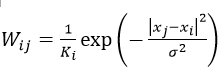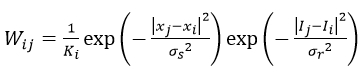## 引导滤波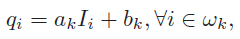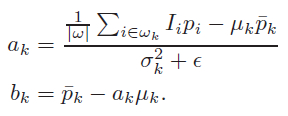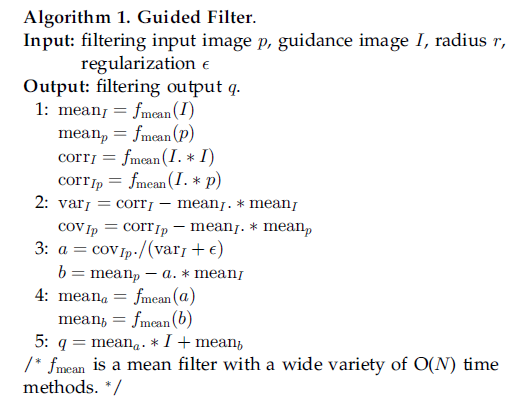[python] view plain
1. def guidedfilter(I,p,r,eps):
2.     '''''I:引导图图；
3.     p:输入图（p=I）;
4.     r :半径:
5.     eps:regulation
6.     f:为窗口半径为r的均值滤波器；
7.     corr：相关；
8.     var：方差；
9.     cov：协方差
10.     '''
11.     height,width = I.reshape()
12.     m_I = cv2.boxFilter(I,-1,(r,r))  #f_mean(I) 均值滤波blur和盒式滤波一样
13.     m_p = cv2.boxFilter(p,-1,(r,r))  #f_mean(p)
14.
15.
16.     m_II = cv2.boxFilter(I*I,-1,(r,r)) #f_mean(I.*I)
17.     m_Ip = cv2.boxFilter(I * p, -1, (r, r))  #f_mean(I.*p)
18.
19.     var_I = m_II-m_I*m_I   #求方差：corr_I -mean_I.*mean_I
20.     cov_Ip = m_Ip - m_I * m_p  #协方差： #cov_Ip-mean_I.*mean_p
21.
22.     a = cov_Ip/(var_I+eps)  #cov_Ip./(var_I+eps)
23.     b = m_p-a*m_I   #mean_p -a.*mean_I
24.     m_a = cv2.boxFilter(a,-1,(r,r))  #mean_a
25.     m_b = cv2.boxFilter(b,-1,(r,r))  #mean_b
26.     return m_a*I+m_b

## 图像处理-小波变换

2019-05-01 15:51:58 qq_30815237 阅读数 13365
• ###### MATLAB图像处理

介绍MATLAB提供的图像处理功能，包括图像基础知识、图像合成、空间变换、邻域和块处理、局部滤波、正交变换、数学形态学、图像分析、图像增强、图像恢复、图像分割、图像配准和图像三维重建等内容。

47课时 342分钟 232人学习 苏金明
免费试看

小波指的是一种能量在时域非常集中的波，它的能量有限，都集中在某一点附近，而且积分的值为零，这说明它与傅里叶波一样是正交波。

图像的傅里叶变换是将图像信号分解为各种不同频率的正弦波。同样，小波变换是将图像信号分解为由原始小波位移和缩放之后的一组小波。小波在图像处理里被称为图像显微镜，原因在于它的多分辨率分解能力可以将图片信息一层一层分解剥离开来。剥离的手段就是通过低通和高通滤波器

小波变换可以和傅里叶变换结合起来理解。傅里叶变换是用一系列不同频率的正余弦函数去分解原函数，变换后得到是原函数在正余弦不同频率下的系数。小波变换使用一系列的不同尺度的小波去分解原函数，变换后得到的是原函数在不同尺度小波下的系数。不同的小波通过平移与尺度变换分解，平移是为了得到原函数的时间特性，尺度变换是为了得到原函数的频率特性。

1.把小波w(t)和原函数f(t)的开始部分进行比较，计算系数C。系数C表示该部分函数与小波的相似程度。

2.把小波向右移k单位，得到小波w(t-k)，重复1。重复该步骤直至函数f结束.

3.扩展小波w(t)，得到小波w(t/2)，重复步骤1,2.

4.不断扩展小波，重复1,2,3.

### haar小波：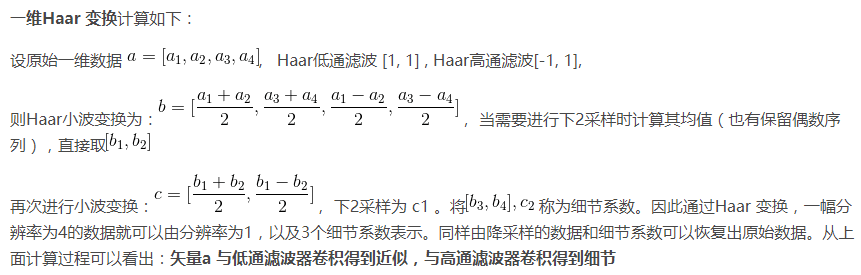### 图像二维离散小波变换 ：

图像的二维离散小波分解和重构过程如下图所示，分解过程可描述为：首先对图像的每一行进行 1D-DWT，获得原始图像在水平方向上的低频分量 L 和高频分量 H，然后对变换所得数据的每一列进行 1D-DWT，获得原始图像在水平和垂直方向上的低频分量 LL、水平方向上的低频和垂直方向上的高频 LH、水平方向上的高频和垂直方向上的低频 HL 以及水平和垂直方向上的的高频分量 HH。

重构过程可描述为：首先对变换结果的每一列进行以为离散小波逆变换，再对变换所得数据的每一行进行一维离散小波逆变换，即可获得重构图像。由上述过程可以看出，图像的小波分解是一个将信号按照低频和有向高频进行分离的过程，分解过程中还可以根据需要对得到的 LL 分量进行进一步的小波分解，直至达到要求。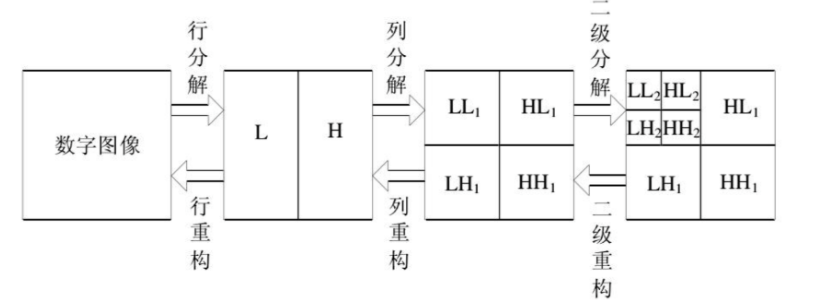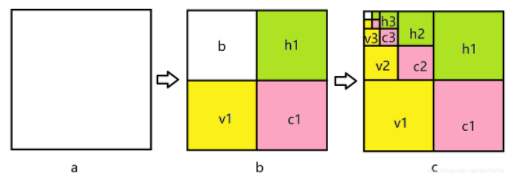# matlab实例

（1）二维信号的小波分解。选择一个小波和小波分解的层次N，然后计算信号s到第N层的分解。
（2）对高频系数进行阈值量化。对于从1~N的每一层，选择一个阈值，并对这一层的高频系数进行软阈值量化处理。
（3）二维小波重构。根据小波分解的第N层的低频系数和经过修改的从第一层到第N的各层高频系数，计算二维信号的小波重构

Matlab函数介绍

1、dwt2是二维单尺度小波变换，其可以通过指定小波或者分解滤波器进行二维单尺度小波分解。DWT2的一种语法格式：[cA,cH,cV,cD]=dwt2(X,'wname')；也就是说DWT2只能对某个输入矩阵X进行一次分解。

[cA1, cH1, cV1, cD1] = dwt2(I_noise, 'haar');
figure
subplot(221), imshow(cA1, []);
subplot(222), imshow(cH1, []);
subplot(223), imshow(cV1, []);
subplot(224), imshow(cD1, []);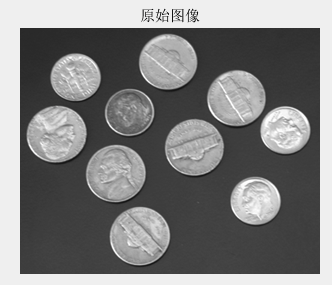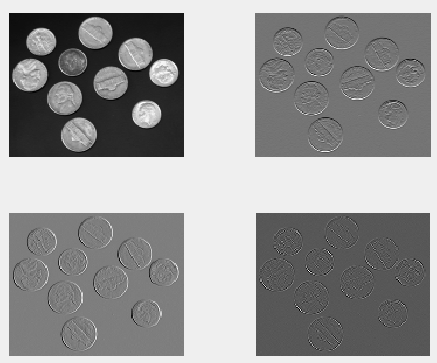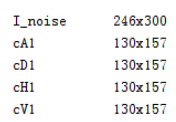2、wavedec2函数

该函数用于对多尺度二维小波进行分解，其常用调用格式：[C,S] = wavedec2(X,N,'wname'):

• X：要进行小波分解的图像；
• N ：指定分解的层数；
• wname：指定用什么小波基进行分解。
• 输出：
• c：为各层分解系数；
• s: 各层分解系数长度，也就是大小。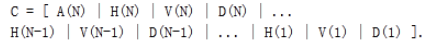C的大小为 [1,img_height×img_width]；A(N)是图像第N层的近似表示，尺度最小，在金字塔中就是每层的下采样的图像，而H、V、D分别表示图像的水平高频分量，垂直高频分量，对角高频分量。正如我们在金字塔概念中所了解的，在第N-1层下采样到N层，N层的图像维度（尺度）是变小了，也就意味着在下采样过程中丢失了信息，而这些丢失的信息实质是高频信息，那么这些信息在小波分解中可以通过HVD这些高频分量来保存。
这里贴上小波分解之后的结果图，直观地感受一下。这里对原始图像进行三层小波分解。红框a表示的就是近似图像。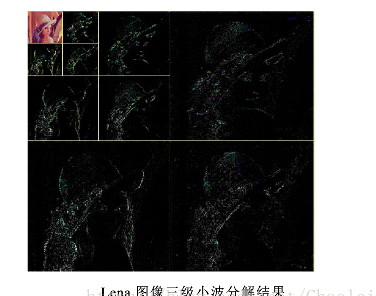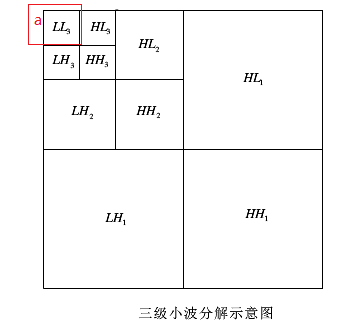需要指出的是，每一次的小波分解都是在近似图像上进行分解。S 是储存各层分解系数长度的,即第一行是A(N)的长度,第二行是H(N)|V(N)|D(N)|的长度,第三行是 H(N-1)|V(N-1)|D(N-1)的长度,倒数第二行是H(1)|V(1)|D(1)长度,最后一行是原始图像img的长度(大小)。 这里原始图像是512×512，并进行了3层的小波分解。对应的s内容如下图：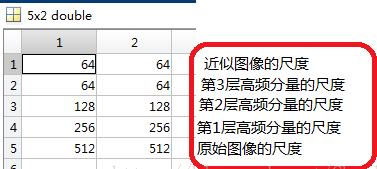S表示每一层分解结果的维数，如果进行n层小波分解，S 的大小是(n+1)*2，最后一行表示的是原始图像的size。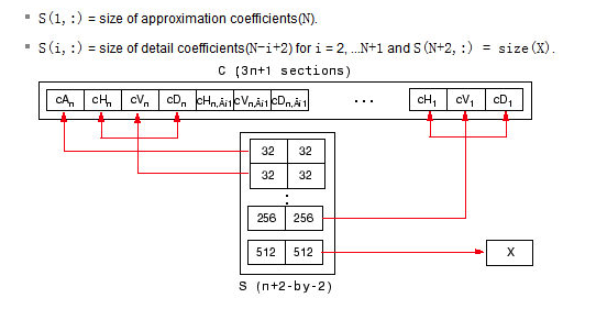3、wdcbm2函数

[thr,nkeep] = wdcbm2(c,s,alpha,m) 返回与level相关的阈值thr和要保持的系数数NKEEP， 函数用于去噪或压缩。使用基于Birge-Massart策略的小波系数选择规则获得thr。通常，alpha= 1.5用于压缩，alpha= 3用于去噪。使用wdcbm2选择各层的独立阈值。

[C，S]是要由wavedec2函数得到的进行去噪或压缩的图像的小波分解结构，level j = size(S,1)-2.

THR是3*j的矩阵，THR（：，j）包含对于level j情况下，水平，对角线和垂直三个方向的阈值。 NKEEP是长度为j的向量，NKEEP（j）包含要保持在级别j情况下系数的数量。

j，M和ALPHA定义策略：

1. 在j + 1级（和更粗略的级别），一切都保留。
2. 对于从1到j的级别i，n_i最大系数保持为n_i = M /（j + 2-i）^ ALPHA。
3. M的默认值是M = prod（S（1，:)）最粗近似系数的数量。

4、wdencmp函数

[XC,CXC,LXC,PERF0,PERFL2] = wdencmp('lvd',C,S,'wname',N,THR,SORH)
函数wdencmp用于一维或二维信号的消噪或压缩。wname是所用的小波函数，gbl(global的缩写)表示每层都采用同一个阈值进行处理，lvd表示每层用不同的阈值进行处理，N表示小波分解的层数，THR为阈值3*N，SORH表示选择软阈值还是硬阈值(分别取为’s’和’h’)，XC是消噪或压缩后的信号，[CXC，LXC]是XC的小波分解结构，PERF0和PERFL2是恢复和压缩L^2的范数百分比, 是用百分制表明降噪或压缩所保留的能量成分。

5、wrcoef2函数

wrcoef2函数是用来重建一幅图像的系数，其实就是根据小波分解之后的系数c来重建其对应的图像。重建好的图像的尺度与原始图像一致。即无论你要重构哪个层的系数，最终它的维度都是和原始图像的尺度一致。其调用形式如下：
X = wrcoef2(‘type’,c,s,’wname’,N)

• type ：指定要进行重构的小波系数，如a –近似图像 ;h – 水平高频分量;v – 垂直高频分量;d–对角高
• 频分量；
• c: 是小波分解函数wavedec2分解的小波系数；
• s: 是wavedec2分解形成的尺度；
• wname ：指定小波基；
• N ：指定重构的小波系数所在的层。 默认重构最大层的系数，N = size(S,1)-2。N所指的层数是如何表示的？比如将图像小波分解成3层，那么N = 3是代表256×256那一层，还是64×64那一层？N=3 代表的是64×64那一层

wrcoef2 的过程就相当于 appcoef2 或者 detcoef2 （抽取系数）后再进行 upcoef2（重构）。

clear;
close all;

file = 'lena_gray_512.tif';
img = double(img);
% 对图像进行3层的小波分解
N = 3; % 设置分解层数
[c,s] = wavedec2(img,N,'db1');

% 对各层的近似图像a进行重构
a1 = wrcoef2('a',c,s,'db1',1);
a2 = wrcoef2('a',c,s,'db1',2);
a3 = wrcoef2('a',c,s,'db1',3);

6、appcoef2 函数

appcoef2适用于2维图像，其主要是为了提取小波分解中形成的近似图像，即低频分量。
A = appcoef2(c,s,’wname’,N)

• c：小波分解的小波系数
• s：小波分解的对应尺度
• wname ：指定小波基
• N ：指定小波系数所在的层数

7、detcoef2

D = detcoef2(O,c,s,N)

• O：指定提取哪个高频分量，取值分别为：’h’ –水平高频 or ‘v’ – 垂直高频 or ‘d’ – 对角高频；
• c：小波系数矩阵；
• s：尺度矩阵；

8、wthcoef2函数

NC = wthcoef2('type',C,S,N,T,SORH):返回经过小波分解结构[C,S]进行处理后的新的小波分解向量NC，[NC,S]即构成一个新的小波分解结构。N是一个包含高频尺度的向量，T是相应的阈值，且N和T长度须相等。返回'type'（水平、垂直、对角线）方向的小波分解向量NC。参数SORH用来对阈值方式进行选择，当SORH = 's'时，为软阈值，当SORH = 'h'时，为硬阈值。

9、重构函数 waverec2

waverec2函数是wavedec2的反函数，返回的结果X就是原始图像。其基于小波分解结构[c,s]对矩阵X进行多级小波重构，其中[c,s]是wavedec2函数的返回值。其调用格式如下：
X = waverec2(c,s,’wname’) )

• c： 系数矩阵
• s： 尺度矩阵
• wname ： 指定小波基

a = (x1+x2)/2 ；d=(x1-x2)/2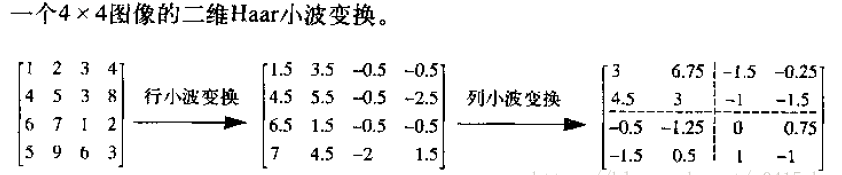## 基于小波变换的图像去噪matlab仿真

2016-05-02 16:18:14 liaozali9022 阅读数 16382
• ###### MATLAB图像处理

介绍MATLAB提供的图像处理功能，包括图像基础知识、图像合成、空间变换、邻域和块处理、局部滤波、正交变换、数学形态学、图像分析、图像增强、图像恢复、图像分割、图像配准和图像三维重建等内容。

47课时 342分钟 232人学习 苏金明
免费试看

1、概况

matlab设计流程：

与单纯运用某种自适应算法相比,基于小波分解的自适应滤波算法在收敛速度和稳定性上都有了很大的提高

2、小波变换的基本理论

示意：尺度越大，采用越大的时间窗，尺度越小，采用越短的时间窗，即尺度与频率成反比。

小波变换与傅里叶变换对比的优点：短时傅立叶分析是把一个短时间的窗函数加在信号上，再对这一部分加窗的信号做傅立叶变换，当然，假定了在短时间内信号是平稳的。然后移动窗函数，对整个时间轴上的信号做短时傅立叶分析。但是，又有问题出现了，短时傅立叶分析一旦确定了窗函数，窗函数的形状便不再改变，于是短时傅立叶分析对于信号的时频分辨率也随之确定。

小波变换的物理表现：分解的结果是产生长度减半的两个部分，一个是经低通滤波器产生的原始信号的平滑部分，另一个则是经高通滤波器产生的原始信号细节部分。

不同j所确定的频带是独立的，随j变化相互独立的频带覆盖了整个频率轴，分辨率j反应了频带的位置和带宽把信号分解到一系列相互独立的频带上分辨率j-1时的近似信号=f(x)在分辨率为j时的近似部分+细节部分

3、小波变换在matlab中的使用

[C, S] = wavedec2(x, n, wname); % 对图像进行小波分解

[C,S]的含义C=[A(n)|H(n)|D(n)|………H(1)|V(1)|D(1)]

h ,v ,d 分别反映水平、垂直、对角线方向

S(1,:)=size of approximation coefficients(n)

%读取MATLAB中的名为detfinger的图像  分辨率：512×512

subplot(1,2,1)

imshow(M);           %显示原始图像

title('原始图像')

P1=imnoise(M,'gaussian',0.02);

%加入高斯躁声,方差为0.02的高斯噪声

P2=imnoise(P1,'salt &pepper',0.02);

%同时加入高斯噪声和椒盐躁声

subplot(1,2,2)

imshow(P2);%加入椒盐躁声后显示图像

title('加入高斯椒盐躁声后');

%%对加入高斯噪声的图像进行小波分解

P2=double(P2);

[CA,CH,CV,CD]= dwt2(P2,'db1 ');

y =[CA,CH;CV,CD];

y=uint8(y);

subplot(3,1,3);

imshow(y);

title('小波分解');

4、滤波器的特性

5、如何设计和建立自适应滤波器

自适应滤波器工作原理是:系统能够按照某种算法自动调节权系数,使其实际输出和期望输出的均方误差达到最小值。

自适应滤波器的结构：有FIRIIR两种。由于IIR滤波器存在稳定性的问题,因此一般采用FIR滤波器。由于FIR滤波器横向结构的算法具有容易实现和计算量少等优点,在对收敛速度不是很快的场合,多采用FIR作为自适应滤波器结构。

LMS算法由于其具有计算量小,稳定性好,易于实现等优点,被广泛使用。

LMS算法中均方误差表示为:

（1）自适应算法中步长 μ 的选择：μ 的大小影响算法的收敛速度。  其中，为矩阵的最大特征值μ 取值小，收敛速度慢，计算工作量大，但滤波性能较好。μ 取值大，收敛速度快，计算工作量小，滤波性能较差。但 μ 大到一定值时，收敛速度变化不明显，且取值过大，会造成计算溢出.

(2)自适应滤波器阶数 的影响： N 不可以任意选取，需要根据经验加上实际仿真验证比较才能最终确定.

、参考信号d(n)、误差信号e(n)

e(n)y(n)d(n)的误差信号，根据最小均方误差算法调节自适应滤波器算法的参数，来优化滤波器结构，从而实现尽可能地保留图像固有的信息。

LMS算法最核心的思想是用平方误差代替均方误差,基本的LMS算法为:

w(k,n+ 1)=w(k,n)+ 2μe(n)x(n-k)

… ,N- 1,N为滤波器的阶数；μ为收敛因子；e(n)=d(n)-y(n)=d(n)-xT(n)w(n)=d(n)-wT(n)x(n)为误差信号；x(n-k)为输入信号；y(n)=xT(n)w(n)为输出信号

6、matlab仿真

%%对加入高斯噪声的图像进行小波分解

J=double(J);

[CA,CH,CV,CD]= dwt2(J,'db1 ');

% [c,s]=wavedec2(j,1,'haar');

y =[CA,CH;CV,CD];

y=uint8(y);

subplot(2,2,3);

imshow(y);

title('小波分解');

%xx=wiener2(CA,[3 3]); %对加噪图像进行二维自适应维纳滤波   %滤波器窗口大小

yy=wiener2(CH,[3 3]); %对加噪图像进行二维自适应维纳滤波   %滤波器窗口大小

zz=wiener2(CV,[3 3]); %对加噪图像进行二维自适应维纳滤波   %滤波器窗口大小

qq=wiener2(CD,[3 3]); %对加噪图像进行二维自适应维纳滤波   %滤波器窗口大小

%%%图像重构

XX = idwt2(CA,yy,zz,qq,'db1');

XX=uint8(XX);

subplot(2,2,4);

imshow(XX);

title('重构以后的图像');

6自适应滤波器的组合设计：

但是当信号源中混有多种干扰噪声时,单独一个滤波器不能达到抵消多种干扰噪声的要求。针对信号源中混有的多种干扰噪声问题,提出了自适应滤波器的组合设计问题。

(不选)      基于小波分解重构的自适应滤波算法比仅进行小波分解的自适应滤波算法滤波精度要稍高一些,但由于需要信号重构,算法的复杂度随之增加,因此该算法实时性相对稍差。

## [Python图像处理] 四.图像平滑之均值滤波、方框滤波、高斯滤波及中值滤波

2018-09-02 23:27:36 Eastmount 阅读数 23615
• ###### MATLAB图像处理

介绍MATLAB提供的图像处理功能，包括图像基础知识、图像合成、空间变换、邻域和块处理、局部滤波、正交变换、数学形态学、图像分析、图像增强、图像恢复、图像分割、图像配准和图像三维重建等内容。

47课时 342分钟 232人学习 苏金明
免费试看

PS：请求帮忙点个Star，哈哈，第一次使用Github，以后会分享更多代码，一起加油。

1.图像平滑
2.均值滤波
3.方框滤波
4.高斯滤波
5.中值滤波

PS：本文介绍图像平滑，想让大家先看看图像处理的效果，后面还会补充一些基础知识供大家学习。文章参考自己的博客及网易云课堂李大洋老师的讲解，强烈推荐大家学习。

PSS：2019年1~2月作者参加了CSDN2018年博客评选，希望您能投出宝贵的一票。我是59号，Eastmount，杨秀璋。投票地址：https://bss.csdn.net/m/topic/blog_star2018/index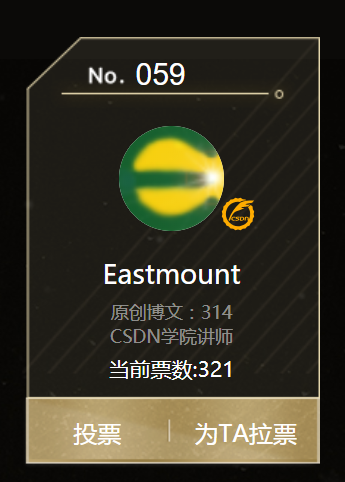# 一.图像平滑

1.图像增强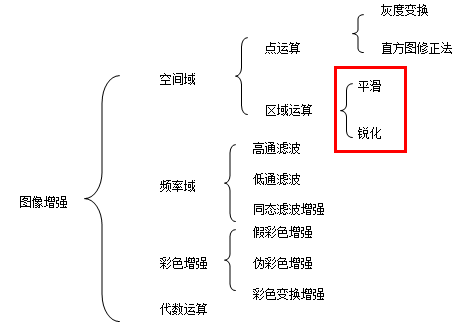2.图像平滑

3.邻域平均法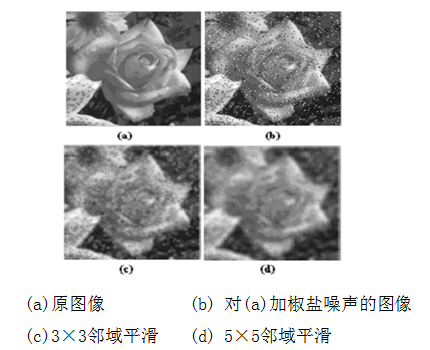# -*- coding:utf-8 -*-
import cv2
import numpy as np

#读取图片
rows, cols, chn = img.shape

#加噪声
for i in range(5000):
x = np.random.randint(0, rows)
y = np.random.randint(0, cols)
img[x,y,:] = 255

cv2.imshow("noise", img)

#等待显示
cv2.waitKey(0)
cv2.destroyAllWindows()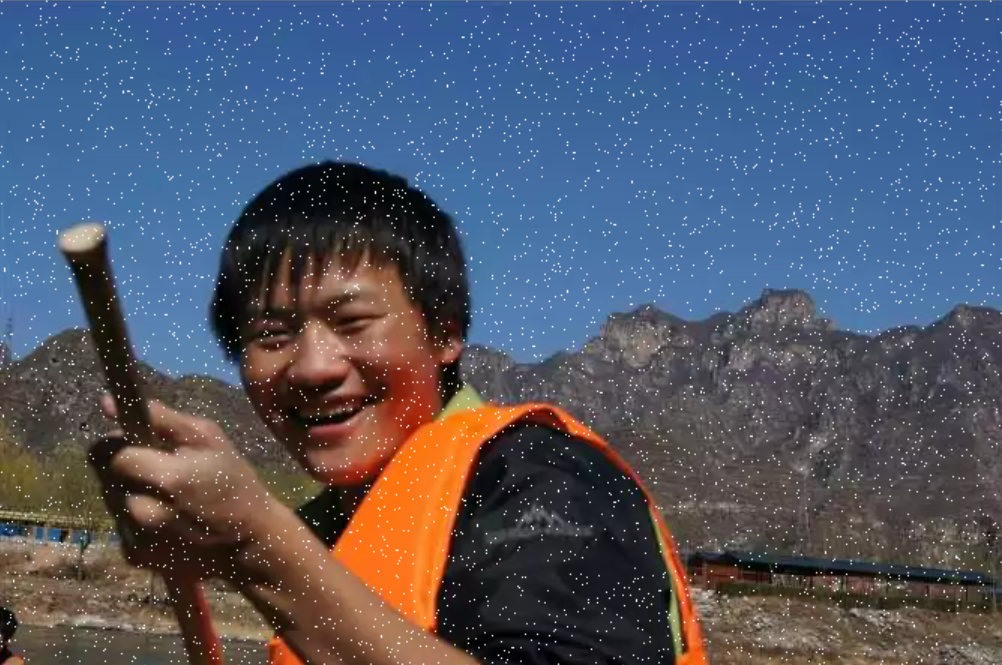# 二.均值滤波

1.原理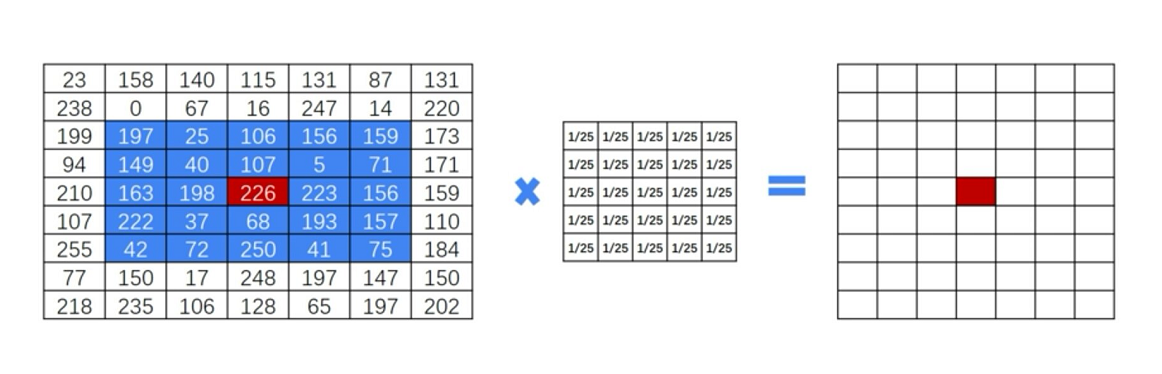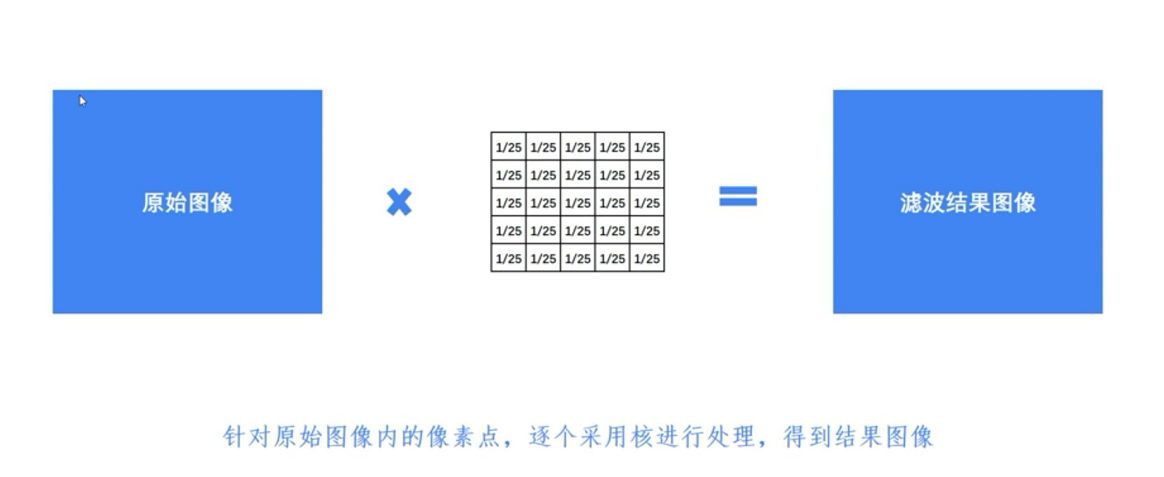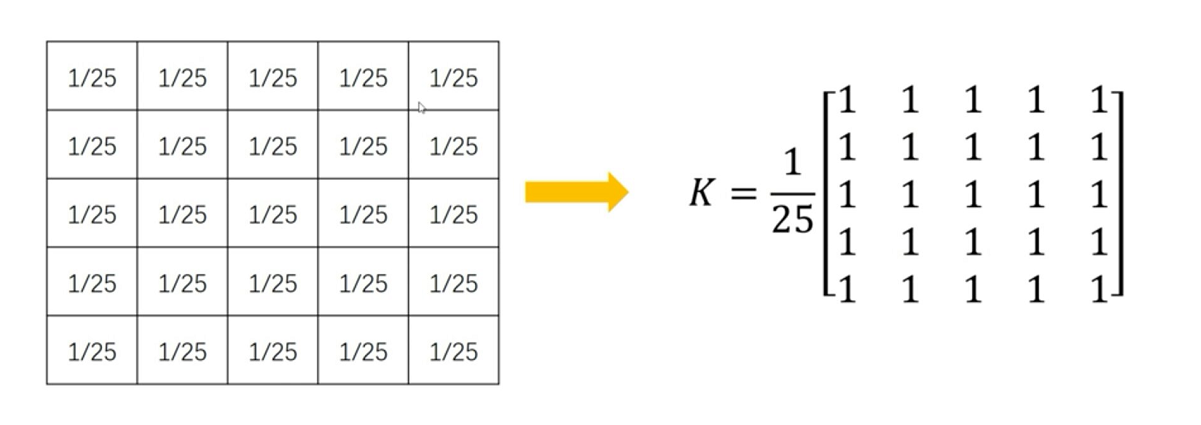2.代码
Python调用OpenCV实现均值滤波的核心函数如下：
result = cv2.blur(原始图像,核大小)

$K=\frac{1}{9}\left[ \begin{matrix} 1 & 1 & 1 \\ 1 & 1 & 1 \\ 1 & 1 & 1 \end{matrix} \right] \tag{1}$
$K=\frac{1}{25}\left[ \begin{matrix} 1 & 1 & 1 & 1 & 1 \\ 1 & 1 & 1 & 1 & 1 \\ 1 & 1 & 1 & 1 & 1 \\ 1 & 1 & 1 & 1 & 1 \\ 1 & 1 & 1 & 1 & 1 \\ \end{matrix} \right] \tag{2}$

#encoding:utf-8
import cv2
import numpy as np
import matplotlib.pyplot as plt

#读取图片
source = cv2.cvtColor(img,cv2.COLOR_BGR2RGB)

#均值滤波
result = cv2.blur(source, (5,5))

#显示图形
titles = ['Source Image', 'Blur Image']
images = [source, result]
for i in xrange(2):
plt.subplot(1,2,i+1), plt.imshow(images[i], 'gray')
plt.title(titles[i])
plt.xticks([]),plt.yticks([])
plt.show()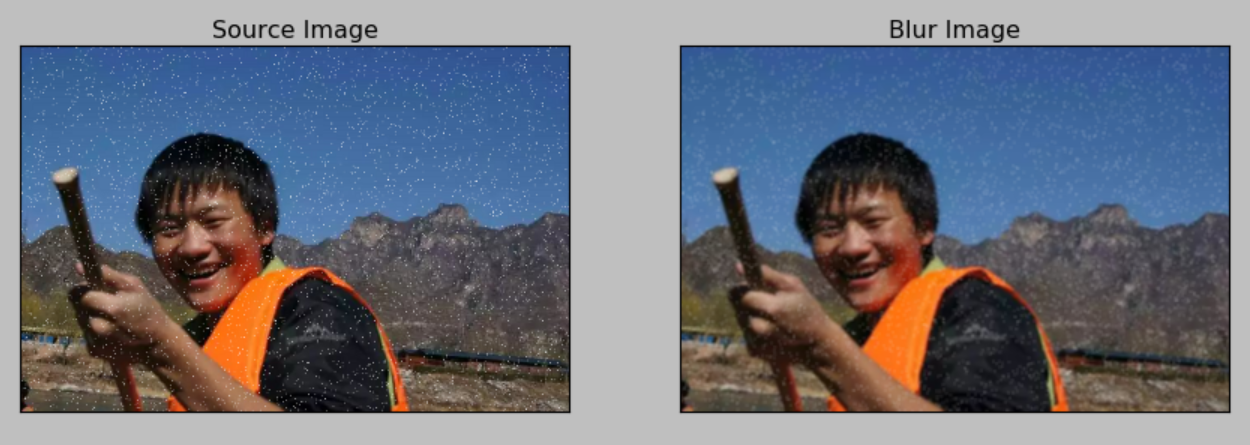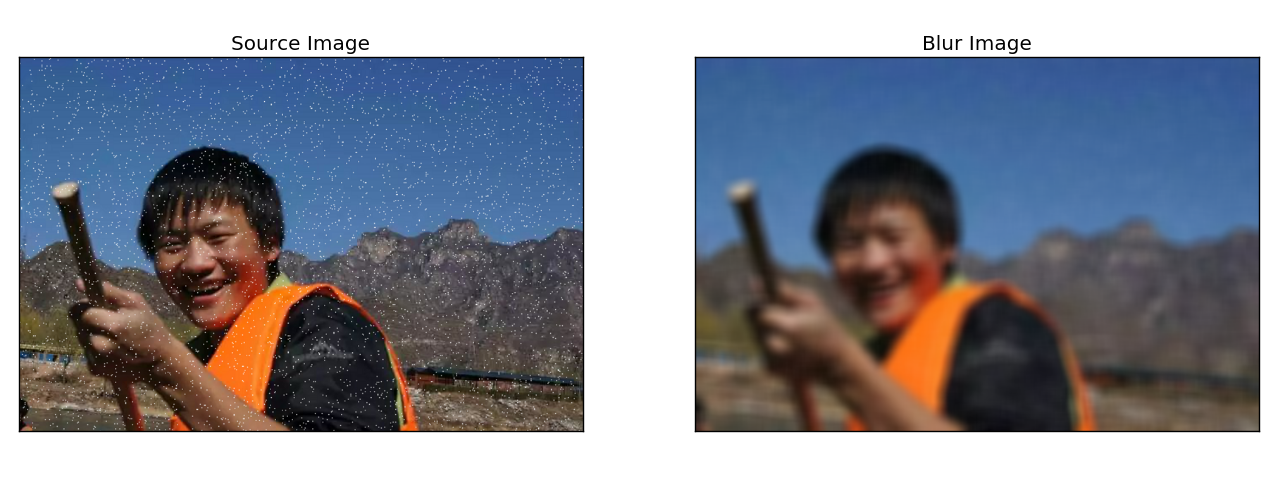# 三.方框滤波

result = cv2.boxFilter(原始图像, 目标图像深度, 核大小, normalize属性)

$K=\frac{1}{9}\left[ \begin{matrix} 1 & 1 & 1 \\ 1 & 1 & 1 \\ 1 & 1 & 1 \end{matrix} \right] \tag{3}$
$K=\frac{1}{25}\left[ \begin{matrix} 1 & 1 & 1 & 1 & 1 \\ 1 & 1 & 1 & 1 & 1 \\ 1 & 1 & 1 & 1 & 1 \\ 1 & 1 & 1 & 1 & 1 \\ 1 & 1 & 1 & 1 & 1 \\ \end{matrix} \right] \tag{4}$

normalize属性表示是否对目标图像进行归一化处理。当normalize为true时需要执行均值化处理，当normalize为false时，不进行均值化处理，实际上为求周围各像素的和，很容易发生溢出，溢出时均为白色，对应像素值为255。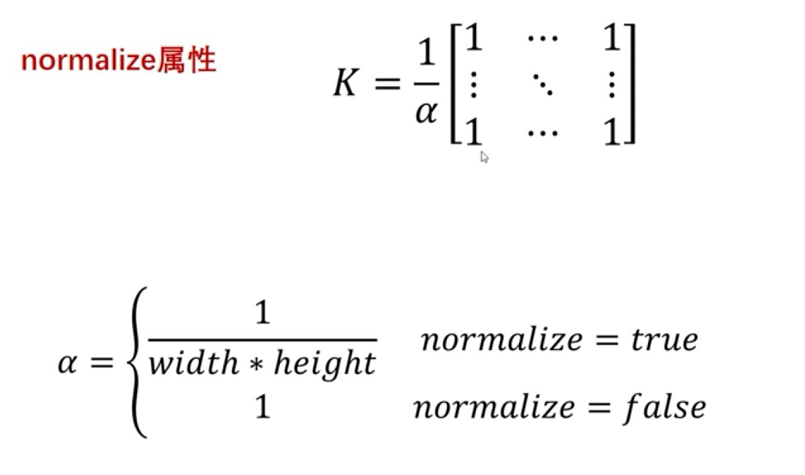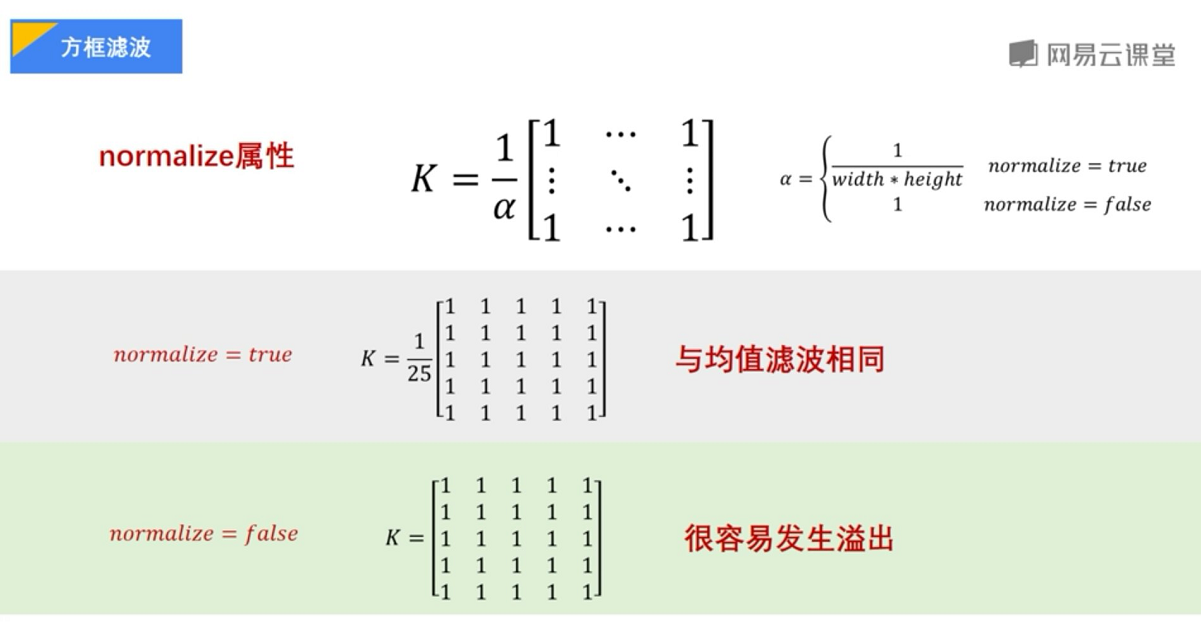#encoding:utf-8
import cv2
import numpy as np
import matplotlib.pyplot as plt

#读取图片
source = cv2.cvtColor(img,cv2.COLOR_BGR2RGB)

#方框滤波
result = cv2.boxFilter(source, -1, (5,5), normalize=1)

#显示图形
titles = ['Source Image', 'BoxFilter Image']
images = [source, result]
for i in xrange(2):
plt.subplot(1,2,i+1), plt.imshow(images[i], 'gray')
plt.title(titles[i])
plt.xticks([]),plt.yticks([])
plt.show()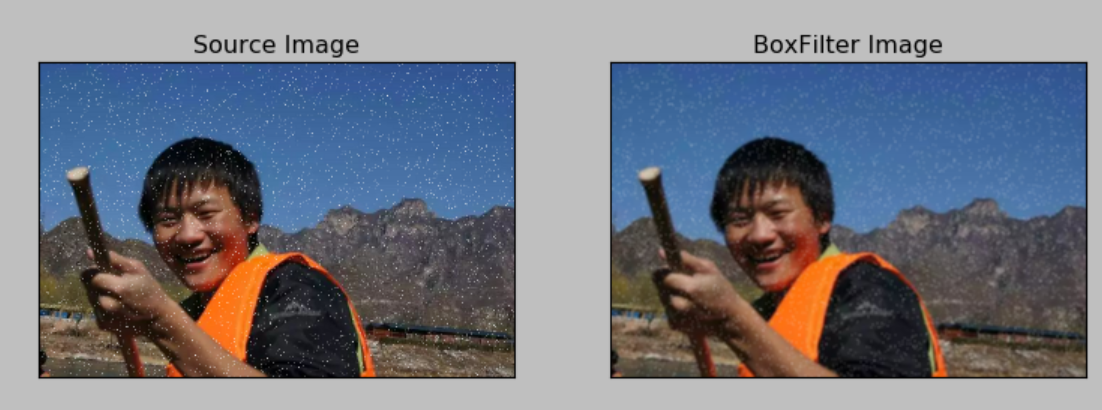print(source[0:3, 0:3, 0])
#[[115 180 106]
# [ 83 152  72]
# [ 55  58  55]]
print(result[0:3, 0:3, 0])
#[[92 90 78]
# [92 89 77]
# [82 80 72]]


#encoding:utf-8
import cv2
import numpy as np
import matplotlib.pyplot as plt

#读取图片
source = cv2.cvtColor(img,cv2.COLOR_BGR2RGB)

#方框滤波
result = cv2.boxFilter(source, -1, (5,5), normalize=0)

#显示图形
titles = ['Source Image', 'BoxFilter Image']
images = [source, result]
for i in xrange(2):
plt.subplot(1,2,i+1), plt.imshow(images[i], 'gray')
plt.title(titles[i])
plt.xticks([]),plt.yticks([])
plt.show()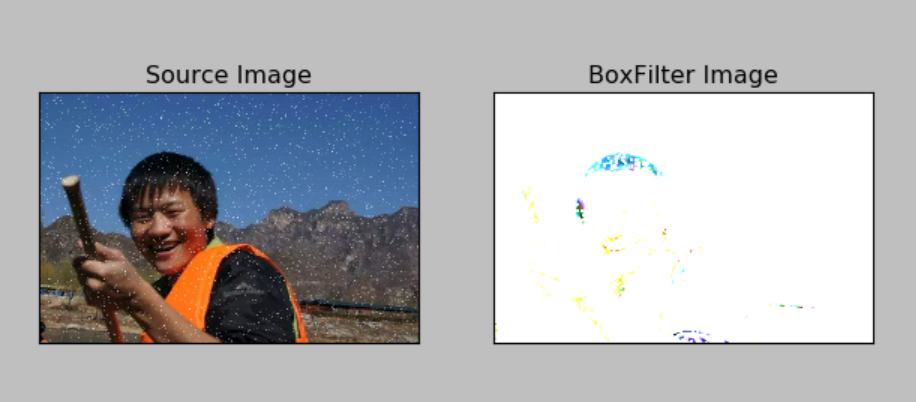result = cv2.boxFilter(source, -1, (2,2), normalize=0)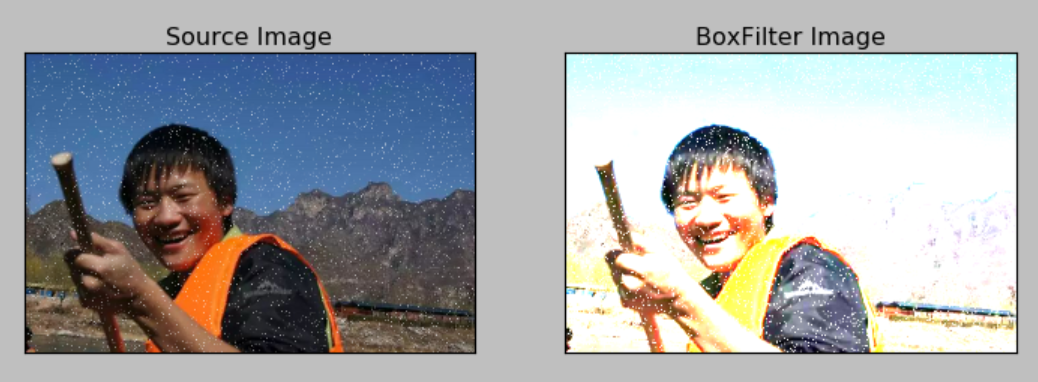# 四.高斯滤波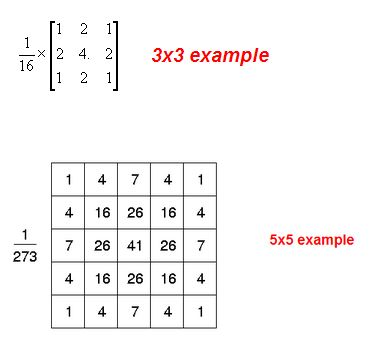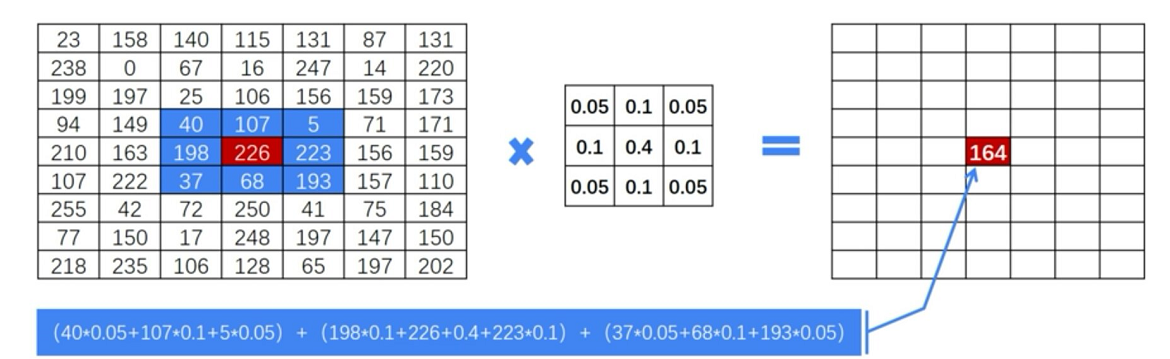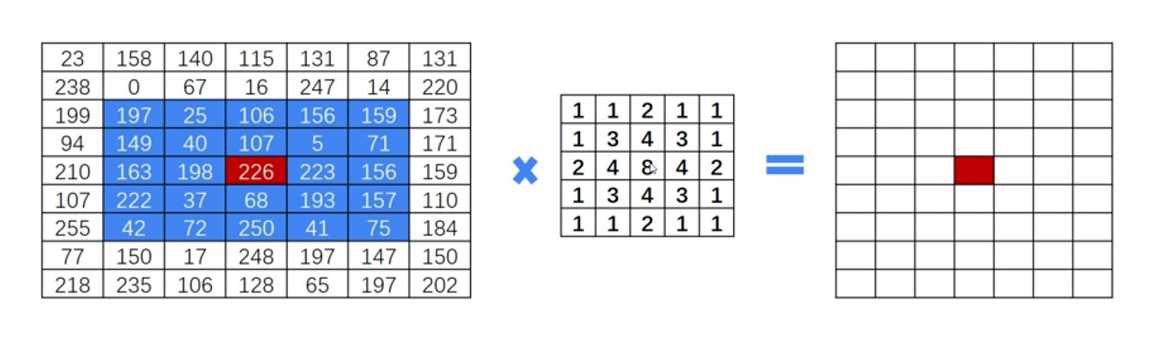Python中OpenCV主要调用GaussianBlur函数，如下：
dst = cv2.GaussianBlur(src, ksize, sigmaX)

$K(3,3)=\left[ \begin{matrix} 0.05 & 0.1 & 0.05 \\ 0.1 & 0.4 & 0.1 \\ 0.05 & 0.1 & 0.05 \end{matrix} \right] \tag{5}$
$K(5,5)=\left[ \begin{matrix} 1 & 1 & 2 & 1 & 1 \\ 1 & 3 & 4 & 3 & 1 \\ 2 & 4 & 8 & 4 & 2 \\ 1 & 3 & 4 & 3 & 1 \\ 1 & 1 & 2 & 1 & 1 \\ \end{matrix} \right] \tag{4}$

#encoding:utf-8
import cv2
import numpy as np
import matplotlib.pyplot as plt

#读取图片
source = cv2.cvtColor(img,cv2.COLOR_BGR2RGB)

#高斯滤波
result = cv2.GaussianBlur(source, (3,3), 0)

#显示图形
titles = ['Source Image', 'GaussianBlur Image']
images = [source, result]
for i in xrange(2):
plt.subplot(1,2,i+1), plt.imshow(images[i], 'gray')
plt.title(titles[i])
plt.xticks([]),plt.yticks([])
plt.show()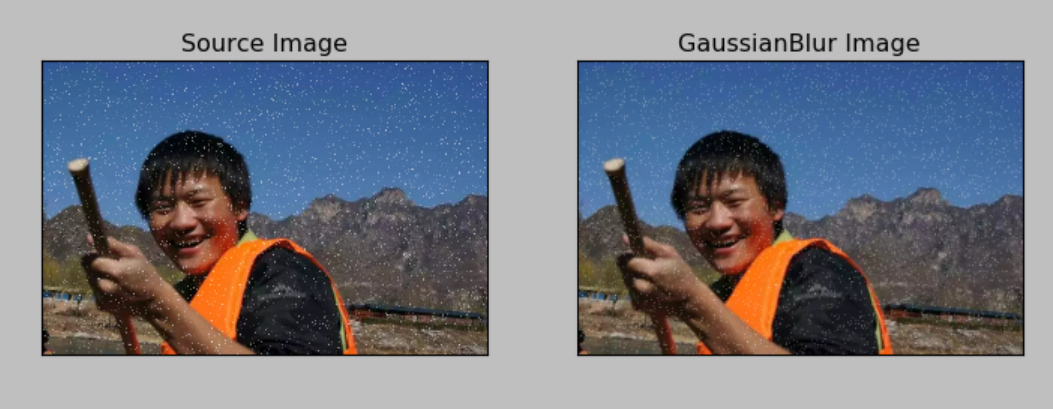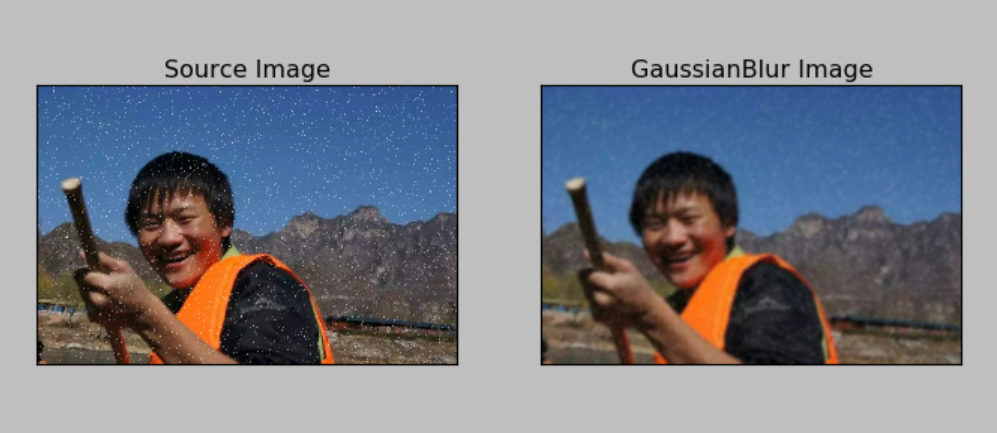# 五.中值滤波

1.概念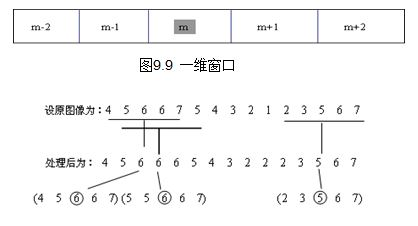2.代码
OpenCV主要调用medianBlur()函数实现中值滤波。图像平滑里中值滤波的效果最好。
dst = cv2.medianBlur(src, ksize)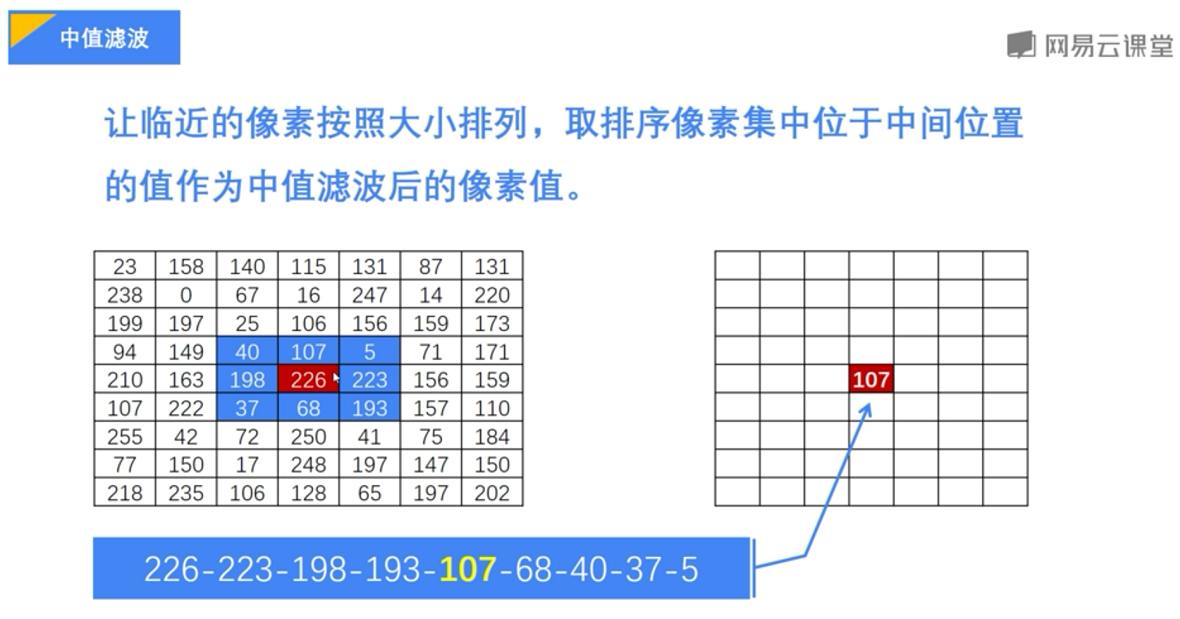#encoding:utf-8
import cv2
import numpy as np
import matplotlib.pyplot as plt

#读取图片

#高斯滤波
result = cv2.medianBlur(img, 3)

#显示图像
cv2.imshow("source img", img)
cv2.imshow("medianBlur", result)

#等待显示
cv2.waitKey(0)
cv2.destroyAllWindows()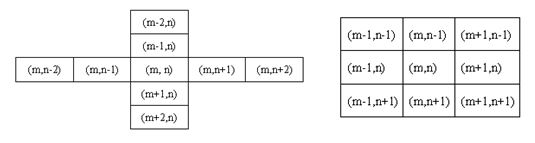（By：Eastmount 2018-09-01 早8点 https://blog.csdn.net/Eastmount/）